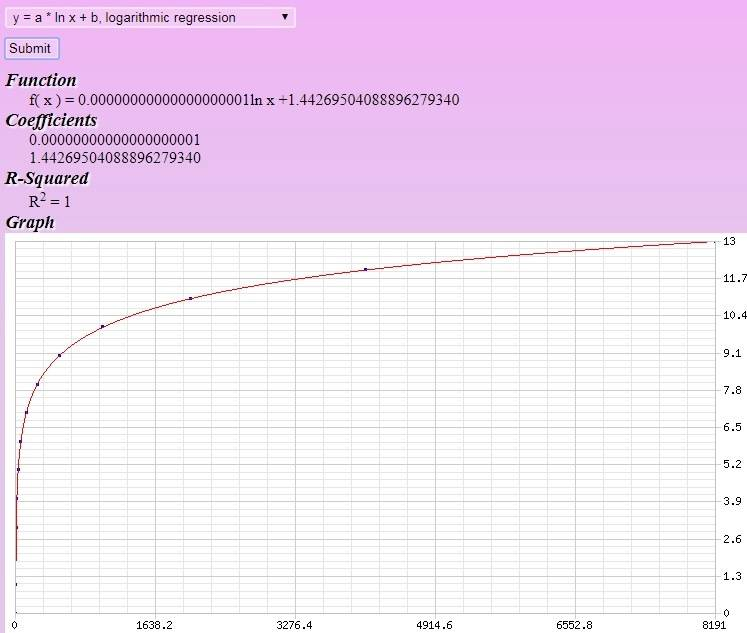# Can this proposition be proved in the Collatz conjecture?

• A
intervoxel
TL;DR Summary
lemma in collatz conjecture proof.
Can this proposition be proved and become a lemma in the proof of Collatz conjecture?

$$collatz(n) \geq \lfloor \frac{log(n)}{log(2)} \rfloor.$$

Looks strange: Collatz conjecture has sequence ending as 1.

•Klystron
SSequence
Looks strange: Collatz conjecture has sequence ending as 1.
I think the symbol "collatz(n)" is supposed to mean:
"the number of steps it takes to reach the value 1, if we are given the starting number n"

So, if we assume that, then the given inequality (or something very close) should be true (in an elementary way) ... but one of the following must be true for that to hold:
(1) We either assume the conjecture to be true (all numbers starting from any "n" go back to 1).
(2) We declare/mention that our inequality is only supposed to hold for those value of "n" for which the calculation stops (goes back to 1) eventually.

Last edited:
Staff Emeritus
You can't tell if something is a lemma without having a proof.

If this isn't obvious, you don't know if something is true or not until you have a proof, so how could you know whether something is a lemma or not?

•fresh_42
Mentor
2022 Award
You can't tell if something is a lemma without having a proof.
And the need for guesswork doesn't help either.
I think the symbol "collatz(n)" is supposed to mean:
"the number of steps it takes to reach the value 1, if we are given the starting number n"

intervoxel
You can't tell if something is a lemma without having a proof.

If this isn't obvious, you don't know if something is true or not until you have a proof, so how could you know whether something is a lemma or not?
I'm not claiming this is a lemma, my friend. I'm asking whether it can be proven, since my attempts to prove it were of no avail. That's why I launched the challenge.

By the way, the formula was deduced by picking the lowest stop times from 1 to 10000 and checking the peculiar power of two sequence that regression fit against $$a\thinspace log(x)+b$$ led to the shown formula.
1,0
2,1
4,2
8,3
16,4
32,5
64,6
128,7
256,8
512,9
1024,10
2048,11
4096,12
8192,13Obs.: a and b are interchanged in the site. A small bug.

Staff Emeritus
Summary: lemma in collatz conjecture proof.

Can this proposition be proved and become a lemma

I'm not claiming this is a lemma, my friend. I'm asking whether it can be proven,

What ever.

$$\frac{\log n}{\log 2} < x+1\leq Collatz(n)$$